## Geodesic

Given two points on a surface, the geodesic is defined as the shortest path on the surface connecting them. Geodesics have many interesting properties. The Normal Vector to any point of a Geodesic arc lies along the normal to a surface at that point (Weinstock 1974, p. 65).

Furthermore, no matter how badly a Sphere is distorted, there exist an infinite number of closed geodesics on it. This general result, demonstrated in the early 1990s, extended earlier work by Birkhoff, who proved in 1917 that there exists at least one closed geodesic on a distorted sphere, and Lyusternik and Schnirelmann, who proved in 1923 that there exist at least three closed geodesics on such a sphere (Cipra 1993).

For a surface given parametrically by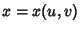,, and, the geodesic can be found by minimizing the Arc Length(1)

But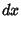(2)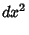(3)

and similarly for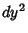and. Plugging in,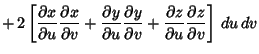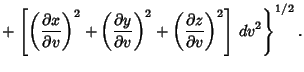(4)

This can be rewritten as(5)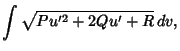(6)

where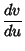(7)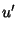(8)

and(9)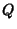(10)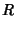(11)

Taking derivatives,(12)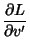(13)

so the Euler-Lagrange Differential Equation then gives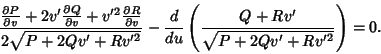(14)

In the special case when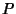,, andare explicit functions ofonly,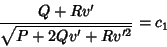(15)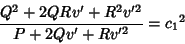(16)(17)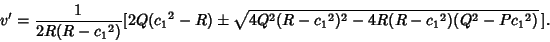(18)

Now, ifandare explicit functions ofonly and,(19)

so(20)

In the casewhereandare explicit functions ofonly, then(21)

so(22)(23)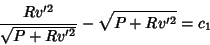(24)(25)(26)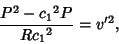(27)

and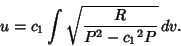(28)

For a Surface of Revolution in which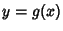is rotated about the-axis so that the equation of the surface is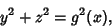(29)

the surface can be parameterized by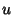(30)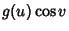(31)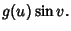(32)

The equation of the geodesics is then(33)

See also Ellipsoid Geodesic, Geodesic Curvature, Geodesic Dome, Geodesic Equation, Geodesic Triangle, Great Circle, Harmonic Map, Oblate Spheroid Geodesic, Paraboloid Geodesic

References

Cipra, B. What's Happening in the Mathematical Sciences, Vol. 1. Providence, RI: Amer. Math. Soc., pp. 27, 1993.

Weinstock, R. Calculus of Variations, with Applications to Physics and Engineering. New York: Dover, pp. 26-28 and 45-46, 1974.